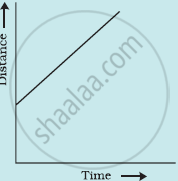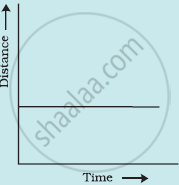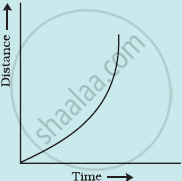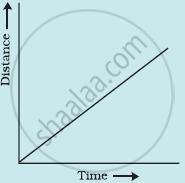CBSE Class 7CBSE
Share

Which of the Following Distance-time Graphs Shows a Truck Moving with Speed Which is Not Constant? - CBSE Class 7 - Science

ConceptDistance-Time Graph

Question

Which of the following distance-time graphs shows a truck moving with speed which is not constant?

••••Solution

Graph (3)

In a distance-time graph, the constant speed of a truck will be represented by a straight line.

In a distance-time graph, a straight line parallel to the time axis indicates that the truck is not moving.

A curved line on the distance-time graph indicates that the truck is moving with a speed which is not constant.

Is there an error in this question or solution?

APPEARS IN

NCERT Solution for Science Textbook for Class 7 (2018 to Current)
Chapter 13: Motion and Time
Q: 13 | Page no. 157
Solution Which of the Following Distance-time Graphs Shows a Truck Moving with Speed Which is Not Constant? Concept: Distance-Time Graph.
S